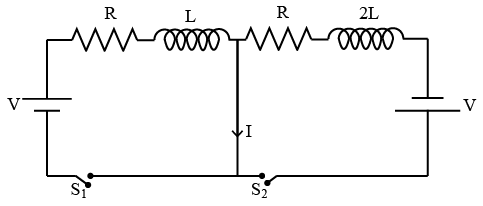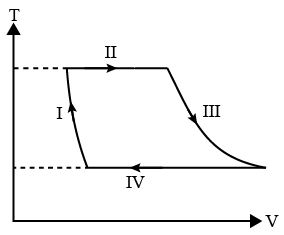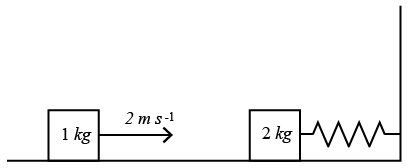Instructions

For the following questions answer them individually

Question 1

# The potential energy of a particle of mass 𝑚 at a distance 𝑟 from a fixed point 𝑂 is given by $$v(r)=\frac{kr^{2}}{2}$$where 𝑘 is a positive constant of appropriate dimensions. This particle is moving in a circular orbit of radius 𝑅 about the point 𝑂. If 𝑣 is the speed of the particle and 𝐿 is the magnitude of its angular momentum about 𝑂, which of the following statements is (are) true?where 𝑘 is a positive constant of appropriate dimensions. This particle is moving in a circular orbit of radius 𝑅 about the point 𝑂. If 𝑣 is the speed of the particle and 𝐿 is the magnitude of its angular momentum about 𝑂, which of the following statements is (are) true?Question 2

# Consider a body of mass 1.0 𝑘𝑔 at rest at the origin at time 𝑡 = 0. A force $$\overrightarrow{F}=(\alpha t \hat{i}+\beta \hat{j})$$ is applied on the body, where $$\alpha=1.0 N S^{-1}$$ and $$\beta=1.0$$N The torque acting on the body about the origin at time 𝑡 = 1.0 𝑠 is 𝜏⃗. Which of the following statements is (are) true?Question 3

# A uniform capillary tube of inner radius 𝑟 is dipped vertically into a beaker filled with water. The water rises to a height ℎ in the capillary tube above the water surface in the beaker. The surface tension of water is $$\sigma$$ The angle of contact between water and the wall of the capillary tube is $$\theta$$. Ignore the mass of water in the meniscus. Which of the following statements is (are) true?Question 4

# In the figure below, the switches $$𝑆_{1} and 𝑆_{2}$$ are closed simultaneously at t = 0 and a current starts to flow in the circuit. Both the batteries have the same magnitude of the electromotive force (emf) and the polarities are as indicated in the figure. Ignore mutual inductance between the inductors. The current 𝐼 in the middle wire reaches its maximum magnitude $$𝐼_{𝑚𝑎𝑥}$$ at time t = $$\tau$$. Which of the following statements is (are) true?Question 5

# Two infinitely long straight wires lie in the 𝑥𝑦-plane along the lines 𝑥 =$$\pm$$𝑅. The wire located at 𝑥 = $$\pm$$𝑅 carries a constant current $$I_{1}$$ and the wire located at 𝑥 = −𝑅 carries a constant current $$I_{2}$$. A circular loop of radius 𝑅 is suspended with its centre at (0, 0, $$\sqrt{3R}$$) and in a plane parallel to the 𝑥𝑦-plane. This loop carries a constant current 𝐼 in the clockwise direction as seen from above the loop. The current in the wire is taken to be positive if it is in the +$$\hat{J}$$ direction. Which of the following statements regarding the magnetic field $$\overrightarrow{B}$$ is (are) true?Question 6

# One mole of a monatomic ideal gas undergoes a cyclic process as shown in the figure (where V is the volume and T is the temperature). Which of the statements below is (are) true?Instructions

For each question, enter the correct numerical value (in decimal notation, truncated/rounded-off to the second decimal place; e.g. 6.25, 7.00, -0.33, -.30, 30.27, -127.30) using the mouse and the on-screen virtual numeric keypad in the place designated to enter the answer.

Question 7

# Two vectors $$\hat{A} and \hat{B}$$ are defined as $$\hat{A}$$=a $$\hat{i}$$ and $$\overrightarrow{B}=a(\cos \omega t \hat{i}+\sin \omega t \hat{j})$$, where a is a constant and $$\omega=\frac{\pi}{6} rad s^{-1}$$.If $$\mid \overrightarrow{A}+\overrightarrow{B}\mid$$=$$\sqrt{3} \overrightarrow{A}-\overrightarrow{B}$$ at time t = $$\tau$$ for the first time, the value of $$\tau$$, in Seconds, is .................

Backspace
789
456
123
0.-
Clear AllQuestion 8

# Two men are walking along a horizontal straight line in the same direction. The man in front walks at a speed 1.0 $$ms^{-1}$$ and the man behind walks at a speed 2.0 $$ms^{-1}$$ . A third man is standing at a height 12 𝑚 above the same horizontal line such that all three men are in a vertical plane. The two walking men are blowing identical whistles which emit a sound of frequency 1430 𝐻𝑧. The speed of sound in air is 330 $$ms^{-1}$$ . At the instant, when the moving men are 10 𝑚 apart, the stationary man is equidistant from them. The frequency of beats in 𝐻𝑧, heard by the stationary man at this instant, is __________.

Backspace
789
456
123
0.-
Clear AllQuestion 9

# A ring and a disc are initially at rest, side by side, at the top of an inclined plane which makes an angle $$60^\circ$$ with the horizontal. They start to roll without slipping at the same instant of time along the shortest path. If the time difference between their reaching the ground is $$\frac{2-\sqrt{3}}{\sqrt{10}}$$ S then the height of the top of the inclined plane, in meters, is __________. Take $$g = 10 ms^{-2}$$

Backspace
789
456
123
0.-
Clear AllQuestion 10

# A spring-block system is resting on a friction less floor as shown in the figure. The spring constant is 2.0 $$Nm^{-1}$$ and the mass of the block is 2.0 kg. Ignore the mass of the spring. Initially the spring is in an unstretched condition. Another block of mass 1.0 kg moving with a speed of 2.0 $$ms^{-1}$$ collides elastically with the first block. The collision is such that the 2.0 kg block does not hit the wall. The distance, in metres, between the two blocks when the spring returns to its unstretched position for the first time after the collision is _________.Backspace
789
456
123
0.-
Clear AllOR